Mobile QR Code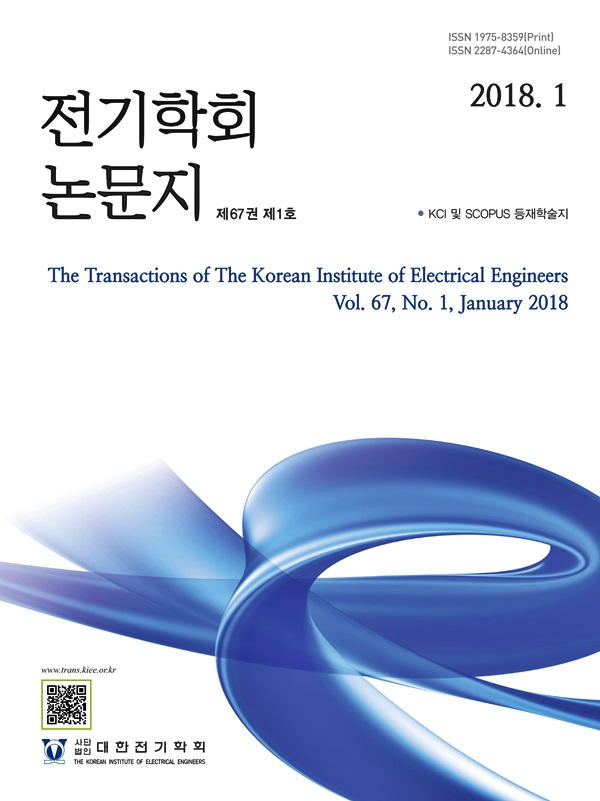The Transactions of the Korean Institute of Electrical Engineers

ISO Journal TitleTrans. Korean. Inst. Elect. Eng.

1. (ESS Sales/Engineering, LG Electronics, Seoul, Korea.)
2. (Dept. of Electrical, Electronics and Communication Engineering, Korea University of Technology and Education, Korea.)

Energy Storage System, Photovoltaic Power Plant, Renewable Energy Generation Forecasting System, Operation Strategy

1. 서 론

According to the 9th Basic Plan for long-term electricity supply and demand of the Korean government, the installed capacity of renewable energy (RE) is expected to be 84.4 [GW] by 2034, which is expected to increase by 65.1 [GW] compared to 2019 (1). When large-scale RE is expanded and supplied to the existing power system, it is necessary to improve the acceptability of RE and to respond to intermittent output charac- teristics such as Photovoltaic Power (PV) and wind power (WP). Accordingly, in January 2021, the Korea Power Exchange (KPX) is conducting a pilot project to introduce RE generation forecasting system (REGFS) to efficiently operate the power system, and the cost for additional start/stop or output increase/ decrease of generators due to RE output change will be reduced (2). In REGFS, small power brokerage business (recruited 20 [MW] or more of PV/WP of 1MW or less) and PV/WP plant (20 [MW] or more) forecast and submit the amount of RE generation a day in advance. If the forecast error rate (FER) on the same day is below 6 [%], and a maximum of 4 won per 1 [kWh] will be paid (3). Various studies have been conducted on REGFS. AI-based power generation and PV prediction model based on specific information such as the temperature, charac- teristics, and local inclination angle of PV module was developed to participate in REGFS (4-5). In addition, in order to reduce the PV output FER, PV output prediction method based on ensemble learning and weather information is presented (6-7).

Meanwhile, ESS (Energy Storage System) is a key facility to solve the intermittent output characteristics of WP/PV power plants. However, the operation purpose of the ESS installed in PV is revenue through the Renewable Energy Certificate (REC) weight given to the amount of discharging. At this time, the ESS shows a simple charging/discharging pattern and the charging time is from 10:00 to 16:00 and the discharging time is from 16:00 to 10:00 of the next day.

Based on the above background, this paper proposes a strategy for securing stable settlement through REGFS participation of PV-linked ESS operators. For example, if the actual PV output on the operating day has a large error compared to the PV output forecasted the previous day, the KPX takes measures to stabilize power supply and demand. In other words, based on the generation planed the previous day, the LNG generator with high fuel cost is ramp up/down for this variation.

Therefore, the new ESS operation strategy is needed to adjust the charging or charging offset so that the PV FER does not exceed ±6 [%] during the ESS charging times (from 10:00 to 16:00). In this paper, it is assumed that the PV prediction accuracy of the previous day is high and the PV FER is maintained within ±10%. Here, ±10% means the minimum value of the monthly average PV FER for the REGFS registration test. Therefore, the FER between ±6% and ±10% can be controlled by adjusting the ESS charging and charging offset. In general, the power management system (PMS) of the ESS can control the output power per second of the Power Conditioning System (PCS) on the day of operation. Therefore, the sum of charging and discharging every 15 minutes can be equal to the expected PV output.

As a result of the simulation under the proposed method, it was confirmed that the PV FER was managed within ±6% and an additional settlement and the existing benefits (SMP(System Marginal Price), REC weight) were secured.

2. General ESS Operational Constraints

2.1 Supply and Demand Conditions

The supply and demand conditions are the same as in Eq. 1. The sum of the PV power output charged and the flowing into the power system during the charging time is equal to the sum of the ESS discharging power and the PV power output flowing into the power system during the discharging time. During ESS discharge, the ESS discharging power supplied to the PV is less than 1%, and it is regarded as 0kW (8).

(1)
$P_{sup}(h)=P_{pv}(h)-P_{cha}(h)+P_{disch}(h)$

where $P_{sup}(h)$ is PV power output per $h$ flowing into the power system during the charging/discharging time, $P_{pv}(h)$ is PV power output per $h$ [kW], $P_{cha}(h)$ is ESS charging power per $h$ [kW], $P_{disch}(h)$ is ESS discharging power per $h$ [kW], $h$ is the time interval of 15 minutes per day

2.2 SOC Operation Conditions

The SOC (state of charge) of the ESS is the same as Eq. 2 that is expressed as the difference between the charging power and the discharging power which the charging/discharging effi- ciency is applied. SOC(1) of the first 15-minute interval ($h$=1) and SOC($n_{h}$) of the last 15-minute interval are assumed to be the same (9).

(2)
$SOC(h)+\dfrac{15}{60}\bullet\left\{\eta\bullet P_{disch}(h)-\dfrac{1}{\eta}\bullet P_{cha}(h)\right\}$ $=SOC(h+1)(1\le h<n_{h})$ $=SOC(1)(h=n_{h})$

where $SOC(h)$ is SOC per $h$ [kWh], $SOC(1)$ is the SOC of the first time interval of the previous day, $\eta$ is ESS charging/discharging efficiency (one-way), $n_{h}$ is total number of 15-minute intervals per day (96=24 hours*4)

2.3 Operating Capacity Limitations

The generation plan of the PV power plant with the ESS is limited by Eq. 3. In particular, the generation plan during the ESS discharging time cannot exceed 70 [%] of PV installed capacity. In addition, the ESS charging power in Eq. 4 should be smaller than the difference between PV power output forecasted the previous day and the charging offset to prevent reverse power during the ESS charging time. The discharging power of the ESS in Eq. 5 is determined within the maximum output range of the PCS, and the SOC is determined within the storage capacity range as in Eq. 6 (10).

(3)
$P_{sup}(h)\le 0.7P_{cap}(h_{DS}\le h\le h_{DE})$

(4)
$P_{cha}(h)\le(P_{pv}(h)-P_{offset})\bullet\mu(h)(h_{CS}\le h\le h_{CE})$

(5)
$P_{disch}(h)\le P_{pcs}\bullet(1-\mu(h))$

(6)
$SOC_{\min}\le SOC(h)\le SOC_{\max}$

where, $P_{cap}$ is PV installed capacity [kW], $h_{DS}$ and $h_{DE}$ are 15 minute time intervals of ESS discharging start and end, $P_{offset}$ is ESS charging offset, $\mu(h)$ is a binary variable representing the ESS operation mode (charging = 1, or discharging = 0), $h_{CS}$ and $h_{CE}$ are 15-minute time intervals of ESS charging start and end, $P_{pcs}$ is maximum output of PCS [kW], $SOC_{\min}$ and $SOC_{\max}$ are minimum and maximum storage capacity of ESS [kWh]

3. ESS Optimal Operation Method for RE Gener- ation Forecasting System

3.1 Objective Function

As shown in Eq. 7, the objective function aims at maximizing the benefits of daily REC weight and REGFS participation for PV-linked ESS operators. In this paper, it is assumed that the actual ESS output [kW] is determined every 1 second, but it is determined in 15-minute units.

(7)
$\max\sum_{ {h}=1}^{ {n}_{ {h}}}\left[C(h)+B_{REF}(h)\right]$

where $C(h)$ is the benefit of ESS charging/discharging for REC weight per $h$ [won], $B_{REF}(h)$ is benefits of participating in REGFS per $h$ [won]

3.2 Benefit for REC Weight

Eq. 8 shows the benefits of REC weight for ESS charging and discharging power. That is, it is calculated by multiplying the sum of PV power output by the settlement unit price (SMP, the REC weighted unit price for PV and ESS): the PV power charged in the ESS during the charging time (10:00~16:00), the discharging power flowing into the power system during the discharging time (17:00~24:00), and the power flowing into the power system during the charging/discharging time(4).

(8)
$C(h)= U_{SMPREC}(h)\bullet P_{su p}(h)\bullet\dfrac{15}{60}$

where $U_{SMPREC}(h)$ is the benefit of REC weight for ESS charging/discharging power per $h$ [won]

3.3 Benefits of Participating in the RE Generation Forecasting System

On the other hand, the benefits generated when participating in the REGFS are expressed as the product of the settlement unit price and the actual PV power output as shown in Eq. 9. In particular, although the settlement unit price is applied according to the FER, the settlement unit price (4 [won/kWh]) applied when the FER is ±6% or less is considered as shown in Eq. 10. The FER of Eq. 11 is calculated as the difference between the PV power output forecasted the previous day and the current day's measured PV power output based on the installed PV capacity (3).

(9)
$B_{REF}(h)= U_{REF}\bullet P_{pv}(h)\bullet 0.25$

(10)
$U_{REF}=4[won/k Wh],\:(0\le FR_{REF}(h)\le 6)$

(11)
$FR_{REF}(h)=\dfrac{\left | P_{fo r}(h)-P_{pv}(h)\right |}{P_{ca p}}\bullet 100$

where $U_{REF}$ is settlement unit price of REGFS [KRW/kWh], $FR_{REF}(h)$ is PV FER per $h$ [%], $P_{fo r}$ is the PV power output forecasted the previous day [kW]

3.4 Constraints on RE Generation Forecasting System

When the FER of RE generation exceeds ±6%, the ESS operation strategy can be divided into two types as follows.

First, if PV power output measured on the day is less than PV power output forecasted the previous day, the measured PV power output with FER of -6% or more in Eq. 11 is reduced by adjusting the charging offset, and the FER kept below 6%. For example, the PV power output reflecting the FER of -6% is calculated based on the PV power output forecasted the previous day as Eq. 12. And when PV power output measured on the day deviates from the result of Eq. 12, the PV power output calculated by Eq. 13 is reflected in the charging offset of Eq. 14. This has the effect of increasing the PV power output flowing into the power system by reducing the PV power output charged in the ESS. In conclusion, the actual PV power output does not exceed the FER of –6%.

(12)
$P_{fo r}^{(-6\%)}(h)=\dfrac{(FR_{REF}^{(-6\%)}\bullet P_{ca p})}{100}+P_{fo r}(h)$

(13)
$P_{dev}^{(-6\%)}(h)=P_{fo r}^{(-6\%)}(h)-P_{pv}(h)$

(14)
$P_{cha}(h)\le(P_{pv}(h)-P_{offset}-P_{dev}^{(-6\%)}(h))\bullet\mu(h)$

where $P_{fo r}^{(-6\%)}(h)$ is PV power output forecasted the previous day with a decrease corresponding to –6% FER per $h$ [kW], $FR_{REF}^{(-6\%)}$ is the FER equivalent to -6% based on PV power output forecasted the previous day, $P_{dev}^{(-6\%)}(h)$ is the difference between the previous day's forecasted PV output with a decrease corresponding to a -6% FER and the actual measured PV output per $h$ [kW]

Second, if the PV power output measured on the day exceeds the PV power output planned the previous day, the PV power output is calculated based on the PV power output planned the previous day as shown in Eq. 15 when the FER is more than +6%. If the PV power output measured on the day deviates from the FER +6%, the increase in the measured PV power output is calculated by Eq. 16. When the constraint of Eq. 17 is reflected, the ESS is charged for the corresponding PV increment so that the FER does not exceed +6%.

(15)
$P_{fo r}^{(+6\%)}(h)=\dfrac{(FR_{REF}^{(+6\%)}\bullet P_{ca p})}{100}+P_{fo r}(h)$

(16)
$P_{dev}^{(+6\%)}(h)=P_{fo r}^{(+6\%)}(h)-P_{pv}(h)$

(17)
$P_{su p}(h)\le P_{fo r}^{(+6\%)}(h)$

where $P_{fo r}^{(+6\%)}(h)$ is the PV power output forecasted the previous day with a decrease corresponding to + 6% FER per $h$ [kW], $FR_{REF}^{(+6\%)}$is the FER equivalent to + 6% based on PV power output forecasted the previous day, $P_{dev}^{(+6\%)}(h)$ is the difference between PV power output forecasted the previous day with a decrease corresponding to a + 6% FER and the actual measured PV output per $h$[kW]

Table 1. Simulation conditions

 Items Parameters PV capacity [kW] 6,000 PCS capacity [kW] 4,000 Battery capacity [kWh] 12,000 $SOC_{\min}$, $SOC_{\max}$ [kWh] 1,200, 12,000 $\eta$ [%] 90 $P_{offset}$ [kW] 400 $P_{pcs}$ [kW] 4,000 $P_{ca p}$ [kW] 4,200 REC weight PV 1 ESS 4 Bidding price REC [won/REC] 50,000 SMP [won/MWh] 90,000

4. Simulation Results and Analysis

The economic feasibility of the proposed ESS charging scheduling was analyzed through comparative analysis of the daily total benefits for Case 1 (existing operation) and Case 2 (proposed operation).

4.1 Simulation Conditions

In order to prove the effectiveness of the proposed ESS charging scheduling, the PV business owner installed PV 6,000 [kW], PCS 4,000 [kW], and Battery 12,000 [kWh], and the depth of discharge (DOD) was 90% and the overall efficiency of the ESS system was 90% as shown in Table 1. The charging offset and PCS maximum output are 400kW and 4,000 [kW], respectively. During ESS discharging, the sum of PV power output and ESS discharging power was limited not to exceed 4,200 [kW], which is 70% of the installed PV capacity.

The REC weights of PV and ESS were selected as 1 and 4, respectively. The charging start and end times were 10:00 and 16:00, and the discharging start and end times were 16:00, and 24:00, respectively.

Meanwhile, to analyze the economic feasibility of the proposed ESS operation strategy, it is assumed that the PV operator participated in the “SMP+1REC” contract market. At this time, 1REC is 40,000 [won], and the SMP unit price per hour is 90,000 [won].

Fig. 1. PV power outputs for simulation (under the FER (±6%))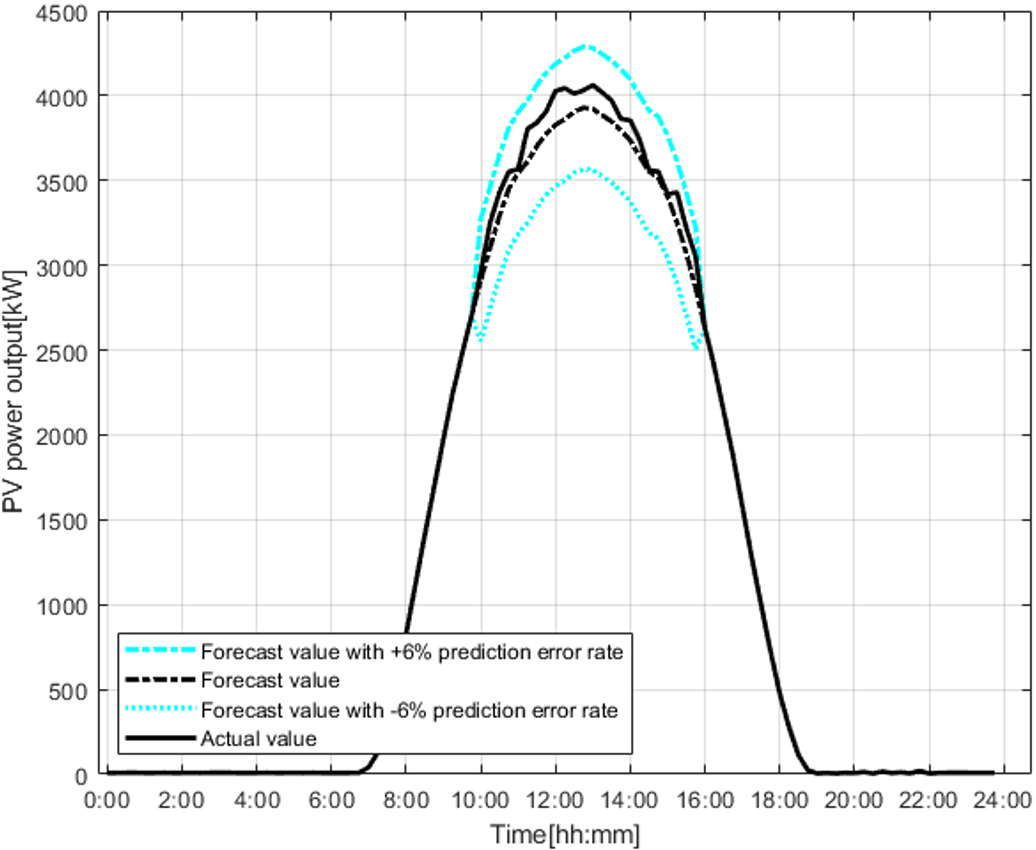Fig. 2. PV power outputs for simulation (over the FER (±6%))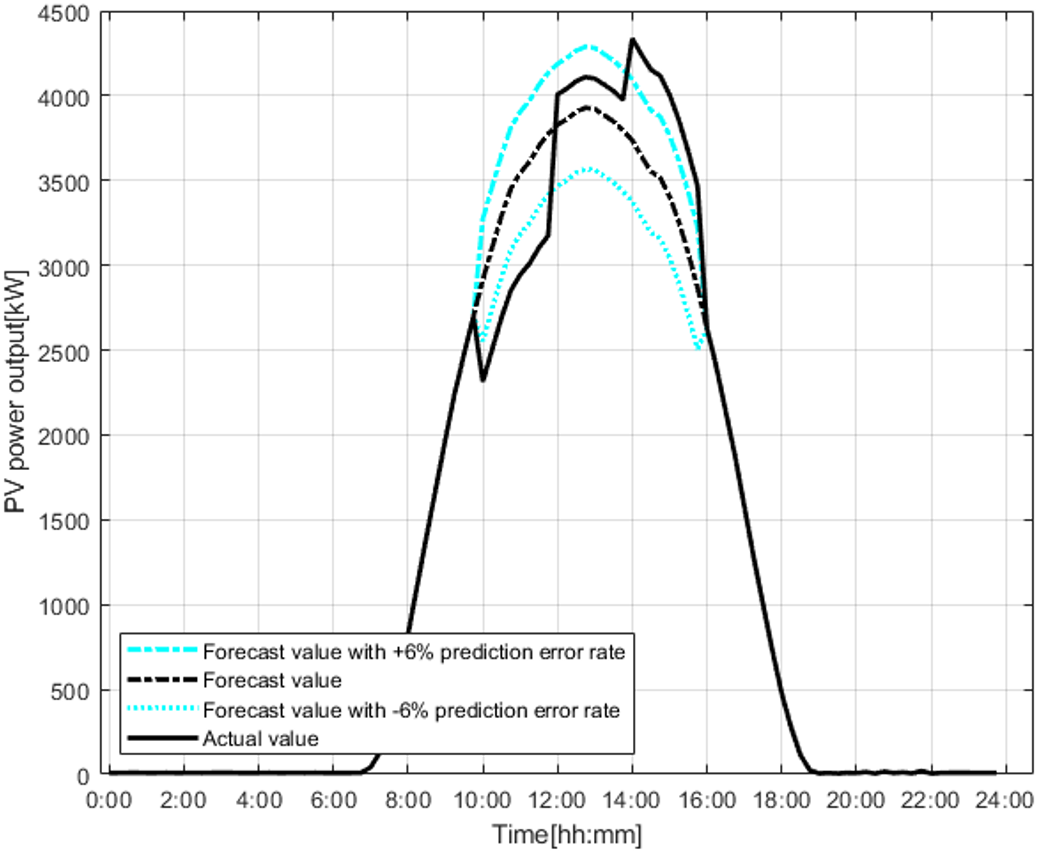Fig. 1 and Fig. 2 show PV power output forecasted the previous day and PV power output the measured on the day for the simulation. Fig. 1 shows that FER is kept within ±6% because PV power output forecasted the previous day (from 10:00 to 16:00) is similar to the PV power output the measured on the day. On the other hand, Fig. 2 shows that the FER is out of ±6%. That is, it is assumed that the output in which the FER (±6%) is reflected based on the PV power output predicted the previous day by Eq. 12 and Eq. 13.

In addition, the PV power output measured on day in Fig. 2 has three characteristics. As a result of comparing the PV power output measured on the day and the PV power output forecasted the previous day, the FER was -10% or less around 10:00~12:00, 3% around 12:00~14:00 and 10% around 14:00~16:00.

4.2 Conventional ESS Charging Scheduling

Fig. 3 shows the simulation results based on Fig. 1. The profit of the PV-linked ESS operator is maximized through the charging and discharging of the ESS. The output obtained by subtracting the charging offset from the PV output is stored in the ESS and the ESS is discharged between 10:00 and 16:00. The power output discharged between 16:00 and 18:30 was limited by Equation (3).

Fig. 3. Conventional ESS charging scheduling ((a) PV power output, (b) Charging power (c) Discharging power (d) SOC)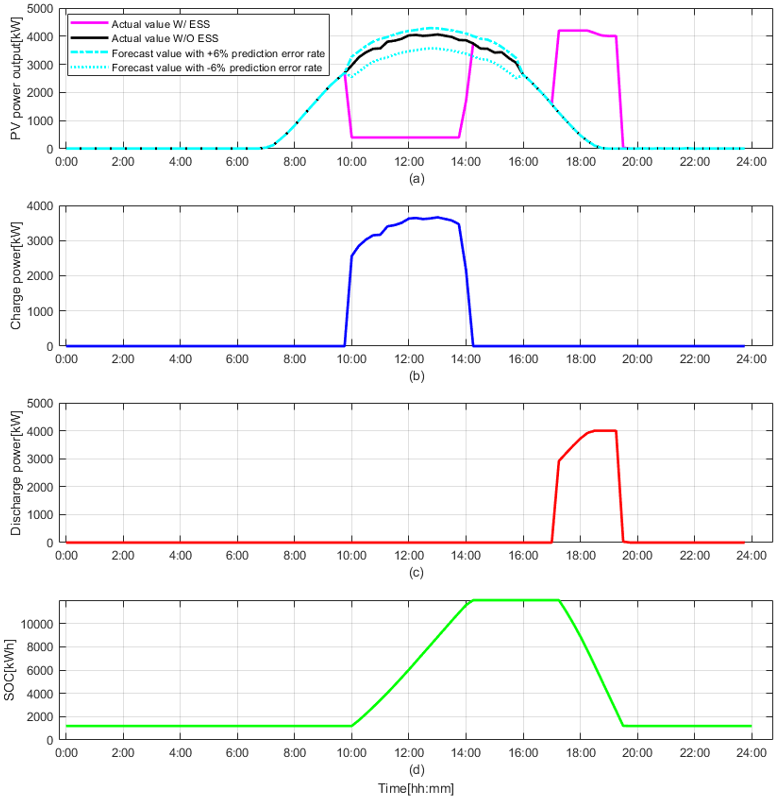4.3 ESS Charging Scheduling for Case 1

Fig. 4 shows ESS charging scheduling when the PV-linked ESS operator participates in the REGFS but the proposed method is not considered. That is, as shown in Fig. 4 (a) and Fig. 4 (b), ESS starts charging at 10:00 and all PV power output are charged after subtracting 400 [kW] which is the charging offset. In Fig. 4 (c), the ESS starts discharging from 17:00, and it is discharged within the range not exceeding 4,200 [kW] which is 70% of the installed PV capacity. Also, ESS charging stopped when SOC reached 90% around 14:30 as shown in Fig. 4 (d). Moreover, the ESS was discharged to 4,000 [kW] which is the maximum output of the PCS, the measured PV power output was 0 [kW] during 18:30~20:00 and the discharging was stopped at 20:00 when the SOC was 3%. As shown in Fig. 4 (a), PV power output the measured on the day compared to PV power output forecasted the previous day has a FER of -10% (600 [kW] reduction) from 10:00 to 12:00 and a FER of +10% (600 [kW] increase) from 14:30 to 16:00, and unsettled payment occurred during that time period.

Fig. 4. ESS charging scheduling for Case 1 ((a) PV power output, (b) Charging power (c) Discharging power (d) SOC)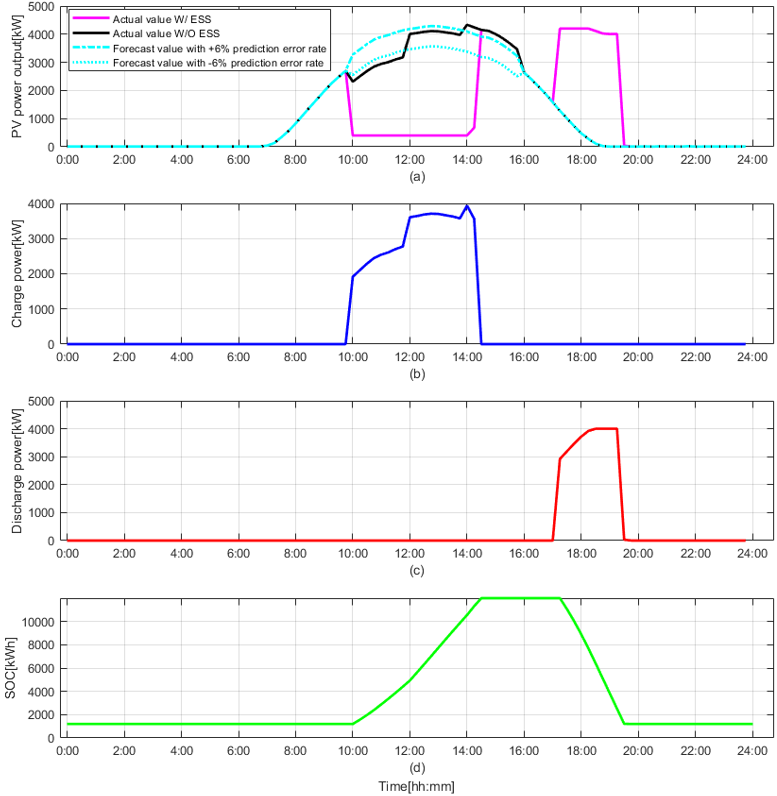Fig. 5. ESS charging scheduling for Case 2 ((a) PV power output, (b) Charging power (c) Discharging power (d) SOC)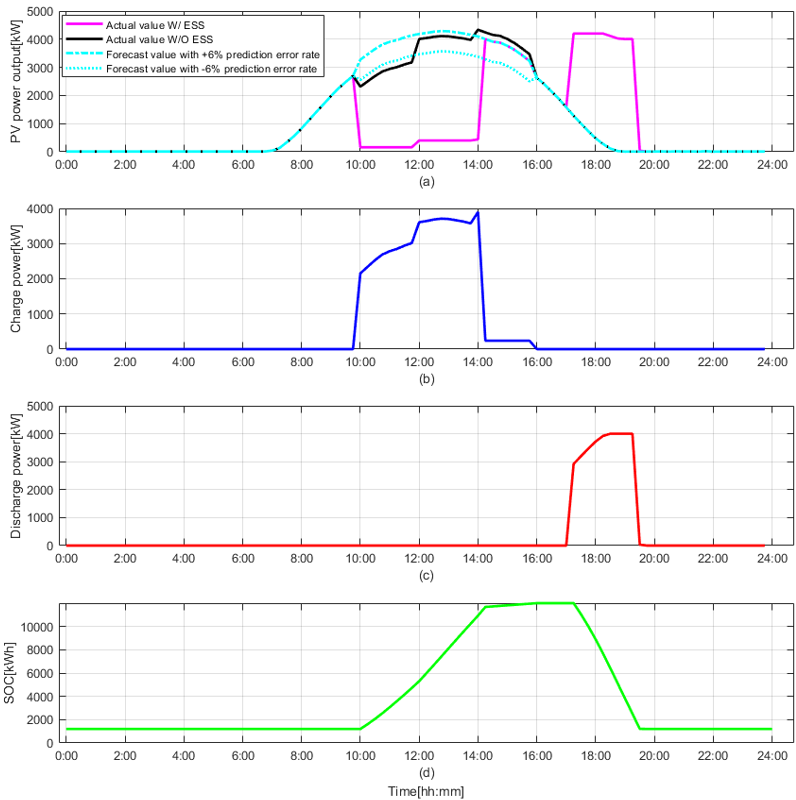4.4 ESS Charging Scheduling for Case 2

Fig. 5 shows the simulation results to which the proposed ESS charging scheduling is applied. The ESS charging scheduling is the same as the simulation result in 4.3 above, however when the PV power output measured on the day has a FER of -10% (600 [kW] reduction) compared to the PV power output forecasted the previous day from 10:00 to 12:00, and the decrease (240 [kW]) calculated by Eq. 13 is reflected in the charging offset. That is, the charging offset was reduced from 400 [kW] to 160 [kW] by Eq. 14. PV power output flowing into the power system was increased (240 [kW]), and the FER was maintained within the range of -6% as shown in Fig. 5(a). When the PV power output measured on the day has a FER of +10% compared to the PV power output forecasted the previous day from 14:30 to 16:00, the increase (240 [kW]) of PV power output was calculated by Equation (16). As shown in Fig. 5(b), the calculated PV increment was charged by Eq. 17 and the FER was maintained in the range of +6%. In conclusion, if it was confirmed that when the PV power output on the day exceeds the ±6% FER, it is possible to stably manage the FER through the charging offset adjustment or the proposed ESS charging scheduling.

Table 2. Economic evaluation results

 Simulation Categories Simulation Cases 1 2 SMP power generation revenue [KRW] 7,154,520 7,154,520 REC power generation revenue [KRW] PV REC weighted revenue 765,000 765,000 ESS REC weighted revenue 1,660,000 1,660,000 REGFS revenue [KRW] 14,400 61,200 Total [KRW] 9,593,920 9,640,720

4.5 Economic Evaluation Results

In order to prove the economic feasibility of the proposed ESS charging scheduling, the daily benefits of the PV-linked ESS were analyzed in an operator's point of view. Daily benefits were analyzed for two cases when the PV-linked ESS operator operated the existing REC weight and REGFS.

⦁ Case 1 (the existing operating method): ESS charging scheduling is determined only by the REC weight.

⦁ Case 2 (the proposed operating method): when the PV power output exceeds the FER of ±6% compared to the PV power output forecasted previous day, the REGFS settlement amount is secured through the charging offset adjustment and ESS charging.

Table 2 shows the economic evaluation results for the above cases. In case 1, the PV generation amount is 15.3 [MWh] and the ESS discharging amount is 8.3 [MWh]. At this time, the SMP revenue is 7,154,520 [won], the PV REC weighted profit is 765,000 [won] and the ESS REC weighted profit is 1,660,000 [won]. In addition, the settlement amount secured through the REGFS is 14,400 [won]. However, unsettled payments were made for the PV power outputs of 6.6 [MWh] in the 10:00 to 12:00 and 5.1 [MWh] in the 14:00 to 16:00, which exceeded the FER of ±6%.

On the other hand, in Case 2, the charging offset was adjusted from 10:00 to 12:00 and the ESS was charged from 14:00 to 16:00 by applying the proposed ESS charging scheduling. Therefore, the FER of the PV power output on the operating day was maintained within ±6% and all settlements have been made. The total settlement obtained through the REGFS was 61,200 [won], which is 46,800 [won] more than in Case 1.

5. Conclusion

The purpose in this paper is to maintain the FER within ±6% when the REGFS is introduced to PV power plant that is currently operated for the purpose of REC weighting. Therefore, the efficient and stable operation is possible from the system side and it is possible to secure stable settlement from the ESS operator side. The main research results are summarized as follows.

(1) By applying the proposed algorithm to 08:00~10:00, which is the charging period of the PV-linked ESS, the ESS can be used to manage the FER when the PV power output on the operating day exceeds the PV power output forecasted the previous day.

(2) When the PV power output deviates from the FER of -6%, the FER was maintained within -6% by compensating for the decrease in the PV power output through adjustment of the charging offset.

(3) When the PV power output deviates from the FER of 6%, the FER was kept within 6% by charging the increment of the PV power output.

(4) When the proposed algorithm was applied to the REGFS, stable benefits were secured by stably maintaining the FER within ±6%.

(5) In terms of power system, the intermittent PV output fluctuations are causing additional operating costs due to real-time power supply. Therefore, the efficient and stable operation of power system is possible by controlling PV volatility through ESS.

Acknowledgements

This work was supported by the 2019 basic research and develop- ment project grant from Korea Electric Power Corporation(KEPCO) (Project No.: R19XO02-02).

References

1
2021, Electricity Supply and Demand Basic Plan, Korea power exchange website. last modified Jan 1, 2021, accessed Nov 5, https://kpx.or.kr/www/contentsdo?key=922
2020, Introduction of Renewable Energy Generation Forecasting System and Energy, Ministry of Trade, Industry and Energy website. last modified Sep 18, 2020, accessed Nov 8, http://www.motie.go.kr/motie/ne/presse/press2/bbs/bbsView.do?bbs_seq_n=163324&bbs_cd_n=813
2020, Distributed Energy Resource Market, Korea power exchange website. last modified Jan 1, 2020, accessed Nov 5, http://der.kmos.kr/sga/mainPage.do4
S. Y. Yang, Y. H. Kim, W. Lee, W. J. Kim, Aug 2021, The Power Brokerage Trading System for Efficient Management of Small-Scale Distributed Energy-Resources, The Journal of the Korea institute of electronic communication sciences, Vol. 16, No. 4, pp. 735-7425
J. J. Song, Y. S. Jeong, S. H. Lee, Mar 2014, Analysis of pre- diction model for solar power generation, Journal of Digital Convergence, Vol. 12, No. 3, pp. 243-2486
Y. R. Lee, W. B. Son, J. L. Kim, 2021, PV Forecasting for Participation in the Distributed Energy Resources Market, the Korean Institute of Electrical Engineers Power Engi- neering Society summer Annual Conf, pp. 521-5227
J. H. Jung, D. J. Kim, G. Y. Sim, 2021, Development of Photovoltaic Power Forecasting Service using Meteorological Information, the Korean Institute of Electrical Engineers Power Engineering Society summer Annual Conf, pp. 2221-22228
J. H. Son, D. S. Rho, M. Y. Kim, Oct. 2021., A Study on Optimal ESS Discharging Schedule for System Marginal Price and Seasonal Peak Demand in Photovoltaic Power Plant, the 9th International Conference on Advanced Power System & Automation & Protection.9
J. H. Son, 2018, A Study on Economic Analysis Algorithm for Energy Storage System Considering Peak Reduction and a Special Tariff, Trans of the KIEE, Vol. 67, No. 10, pp. 1278-128510
J. H. Son, D. S. Rho, M. Y. Kim, Oct. 2021., A Study on Optimal ESS Charging Scheduling Considering Power Gener- ation Prediction in Photovoltaic Power Plant, the 9th International Conference on Advanced Power System & Automation & Protection.저자소개

He received the Ph.D. degree from Hokkaido University, Sapporo, Japan, in 2016.

From December 2016, he has been working as a manager of LG Electronics’ ESS Sales/Engi- neering Team.

His research interests are analysis and operation of power system, optimal operation of distributed generation with ESS and demand response.

He received the Ph.D. degree from Hokkaido University, Sapporo, Japan, in 1997.

From December 1999, he was appointed to his current position as an Professor at Korea University of Technology and Education.

His research interests are analysis and operation of power system, grid connection of distributed generation and power quality

He received the Ph.D. degree from Hokkaido University, Sapporo, Japan, in 1997.

From December 1999, he was appointed to his current position as an Professor at Korea University of Technology and Education.

His research interests are analysis and operation of power system, grid connection of distributed generation and power quality